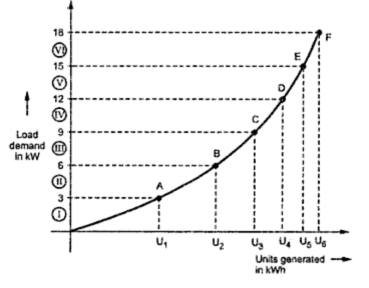A plot of number of units generated (kWh) for a given demand (kW) is called integration load duration curve on Y-axis, load demand in kW or MW is plotted while on z-axis corresponding number of units generated are obtained. Such a curve corresponding to load duration curve shown in Fig1.Fig. 1  Integrated Load Duration Curve

This curve is obtained from load duration curve. Let the load demand be 3 kW from the load duration curve in section I. The number of units generated corresponding to this demand will be area under section I which is shown as U in integrated load duration curve. Similarly the other pints are also obtained to get a total curve.
The number of units consumed by a load upto a particular time of a day can also be shown on a curve which is called as mass curve.